Create an Account

Home / Questions / 1 A parallel LC tank circuit has a Q of 60 and coil winding resistance of 5 n Determine th...

1 A parallel LC tank circuit has a Q of 60 and coil winding resistance of 5 n Determine the circuit&39 s impedance at resonance 2 A parallel LC tank circuit has and a coil winding resislance

1. A parallel LC tank circuit has a Q of 60 and coil winding resistance of 5 n. Determine the circuit&#39;s impedance at resonance.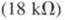2. A parallel LC tank circuit has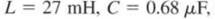and a coil winding resislance of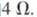Calculate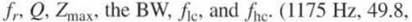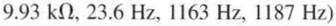Jul 29 2020 View more View LessSubscribe To Get Solution